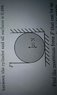# Maximum force to not rotate a cylinder

• nautola
In summary, the question asks for the maximum force that can be applied to a vertical cylinder against a wall without causing it to rotate. After setting up equations, it is determined that there must be a normal force and a friction force acting on the cylinder to prevent it from moving. Using three equations, the maximum force is calculated to be a little less than 3 N.

## Homework Statement

A vertical cylinder is sitting against a wall. It has a weight of 20N and no given radius. A force is applied tangentially in a vertical direction on the side not against the wall. The coefficient of friction is 0.499 for all surfaces. What is the maximum force so that the cylinder will not rotate?

## Homework Equations

τ= Fr-f(bottom)r-f(wall)r=0
f=μN

## The Attempt at a Solution

I set up the necessary equations and I thought that since there wasn't a normal force applied horizontally, the wall didn't have any friction so I neglected it and answered 9.98. That wasn't correct.

#### Attachments

•IMAG0033.jpg
18.2 KB · Views: 303
The wall exerts a normal force and has a friction force. The cylinder isn't moving so sum of torques, and x and y forces equals 0. Draw a free body diagram to account for all the forces.

I might have a solution however I'd rather not give you a possibly incorrect set of reasoning if it turns out to be wrong, care to give the correct number?

I wouldn't call that a "vertical" cylinder!
It is getting very late here, and I usually write nonsense late at night, but perhaps you will find something useful in this argument.
There must be a normal force pressing it against the wall.
Consider the horizontal forces on the cylinder. You have a friction force F₁ on the bottom going to the right (opposing the turning). There must be another horizontal force on the side preventing the cylinder from accelerating to the right - the wall pushes on the cylinder with F₁. (This F₁ results from a normal force F₀ acting down on the floor and up on the cylinder F₁ = μF₀ ). So the cylinder must push on the wall with F₁ . This normal force results in an upward friction force μF₁ . Vertically we have no acceleration so F + μF₁ - mg + F₀ = 0 . The torques about the touch point on the wall add up to zero: F*2r - mgr + F₀r = 0 . We have 3 equations in unknowns F,F₀ and F₁. Solve them for F. I got a little less than 3 N but I usually calculate incorrectly late at night.